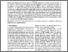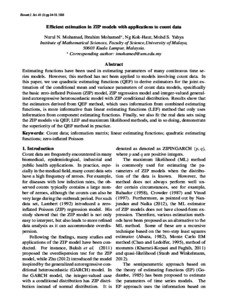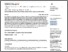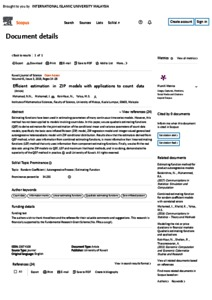# Efficient estimation in ZIP models with applications to count data

Mohamad, Nurul Najihah and Ng, Kok-Haur and Yahya, Mohd S. (2018) Efficient estimation in ZIP models with applications to count data. Kuwait Journal of Science, 45 (3). pp. 14-28. ISSN 1024-8684Preview
PDF (Efficient estimation in ZIP models with applications to count data) - Published VersionPreview
PDF (SCOPUS) - Supplemental Material

## Abstract

Estimating functions have been used in estimating parameters of many continuous time series models. However, this method has not been applied to models involving count data. In this paper, we use quadratic estimating functions (QEF) to derive estimators for the joint estimation of the conditional mean and variance parameters of count data models, specifically the basic zero-inflated Poisson (ZIP) model, ZIP regression model and integer-valued generalized autoregressive heteroscedastic model with ZIP conditional distribution. Results show that the estimators derived from QEF method, which uses information from combined estimating functions, is more informative than linear estimating functions (LEF) method that only uses information from component estimating functions. Finally, we also fit the real data sets using the ZIP models via QEF, LEF and maximum likelihood methods, and in so doing, demonstrate the superiority of the QEF method in practice.

Item Type: Article (Journal) 7795/81076 Count data; information matrix; linear estimating functions; quadratic estimating functions; zero-inflated Poisson Q Science > QA Mathematics Kulliyyah of Science > Department of Computational and Theoretical Sciences Dr Nurul Najihah Mohamad 22 Jul 2020 15:54 22 Jul 2020 15:55 http://irep.iium.edu.my/id/eprint/81076View Item